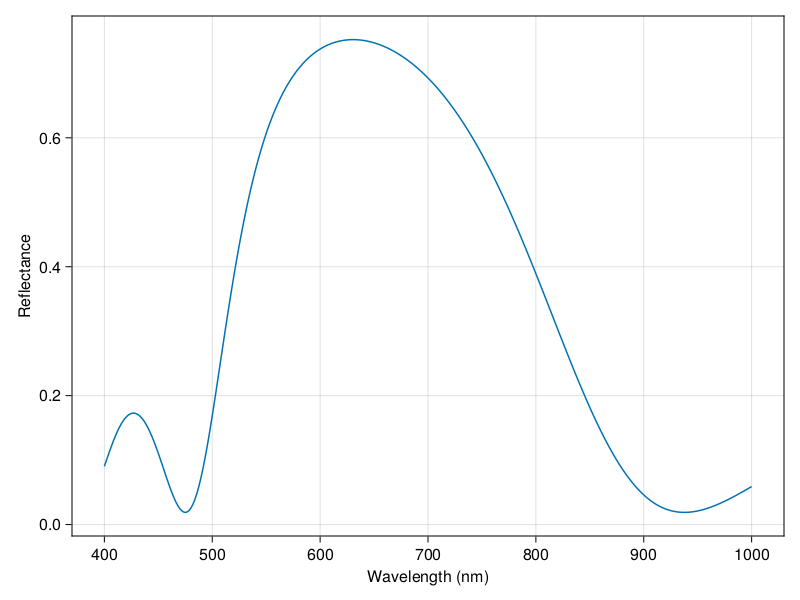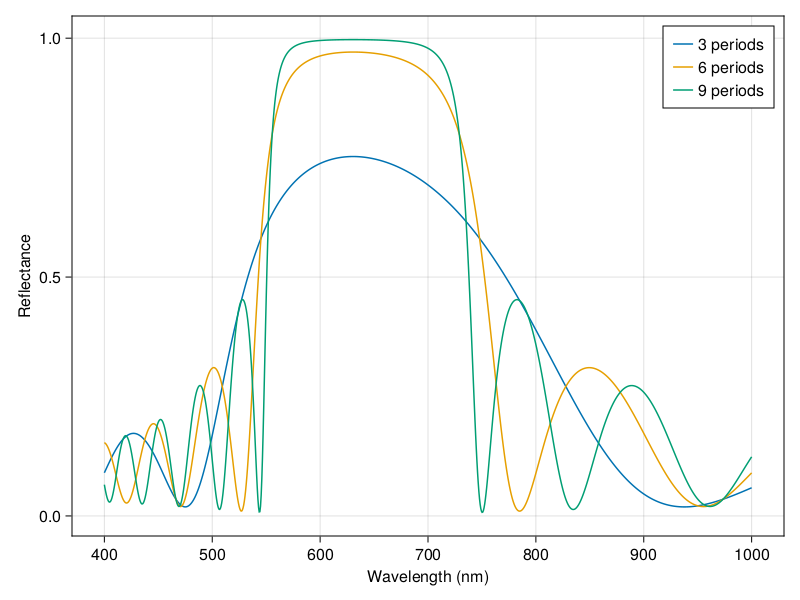# Quick Start

If you just want to get started with a transfer matrix calculation and plot the transmittance or reflectance spectrum, this is the place to start. First make sure to install TransferMatrix.jl by typing ] from the Julia REPL to enter Pkg mode, then run

pkg> add https://github.com/garrekstemo/TransferMatrix.jl.git

## Quarter-wave mirror

Let's make a simple quarter-wave mirror, or distributed bragg reflector (DBR). We will do this via alternating layers of titanium dioxide (n = 2.13) and silica (n = 1.46) optimized for a wavelength of 630 nm. We'll make three periods of these two layers and and layer of air. This 4x4 transfer matrix method simultaneously calculates the transmittance and reflectance for s-polarized and p-polarized radiation. (We are using the powerful plotting library Makie.jl to produce the figures.)

using TransferMatrix
using CairoMakie

λs = collect(range(0.4, 1.0, length = 1000)) .* 1e-6
n_tio2 = fill(2.13, length(λs))
n_sio2 = fill(1.46, length(λs))

air = Layer("Air", 0.5e-6, λs, fill(1.0, length(λs)), zeros(length(λs)))
tio2 = Layer("TiO2", 0.074e-6, λs, n_tio2, zeros(length(λs)))
sio2 = Layer("SiO2", 0.108e-6, λs, n_sio2, zeros(length(λs)))

layers = [air, tio2, sio2, tio2, sio2, tio2, sio2]

s = Structure(layers, λs, [0.0])
Tp, Ts, Rp, Rs = calculate_tr(s)

fig, ax, l = lines(λs .* 1e9, Rp, label = "3 periods")

ax.xlabel = "Wavelength (nm)"
ax.ylabel = "Reflectance"

figNow let's try a few more periods and plot them all together to see how the reflectance changes with increasing number of layers. Notice that we are adding new layers directly to the structure and not creating a new structure.

nperiods = 6

for i in 1:nperiods
push!(s.layers, tio2)
push!(s.layers, sio2)
if i%3 == 0
Tp, Ts, Rp, Rs = calculate_tr(s)
lines!(ax, λs .* 1e9, Rp, label = "\$(i + 3) periods")
end
end

axislegend(ax)
figYou can see that it's quite simple to make a structure and modify the layers. Note that while an individual Layer is immutable, you can modify the properties of a Structure.